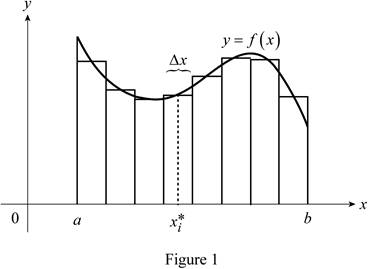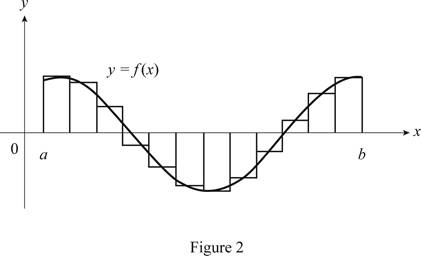Chapter 4, Problem 1RCC

Chapter
Section
Textbook Problem

(a) Write an expression for a Riemann sum of a function f on an interval [a, b]. Explain the meaning of the notation that you use.(b) If f ( x ) ⩾   0 , what is the geometric interpretation of a Riemann sum? Illustrate with a diagram.(c) If f(x) takes on both positive and negative values, what is the geometric interpretation of a Riemann sum? Illustrate with a diagram.

(a)

To determine

To find: The expression for a Riemann sum of a function f.

The expression for a Riemann sum of a function f is i=1nf(xi*)Δx.

Explanation

The Riemann sum of a function f is the method to find the total area underneath a curve.

The area under the curve dividedas n number of approximating rectangles. Hence the Riemann sum of a function f is the sum of the area of the all individual rectangles.

R=i=1nf(xi*)Δx

Here, xi* is a point in the i subinterval [xi1,xi] and Δx is the length of the sub intervals.

Thus, the expression for a Riemann sum of a function f is i=1nf(xi*)Δx.

b)

To determine

To define: The geometric interpretation of a Riemann sum with diagram.

Explanation

Given information:

Consider the condition for the function f(x)0

Explanation:

The function f(x)0 represents that the function is in the first quadrant of the graph.

Sketch the curve f(x) in the first quadrant and then separate the area under the curve with n number approximating rectangles.

Show the curve as in Figure 1.Refer to Figure 1

The function f(x) is positive. Hence the sum of areas of rectangles underneath the curve is the Riemann sum.

Thus, the geometric interpretation of a Riemann sum of f(x)0 is defined.

c)

To determine

To define: The geometric interpretation of a Riemann sum, if the function f(x) takes on both positive and negative values.

Explanation

Given information:

The function f(x) takes on both positive and negative values.

Explanation:

The function f(x) takes on both positive and negative values represents that the function is in the first and fourth quadrant of the graph.

Sketch the curve f(x) in the first and third quadrant and then divide the area under the curve and above the curve with n number approximating rectangles.

Show the curve as in Figure 2.Refer figure 2,

The Riemann sum is the difference of areas of approximating rectangles above and below the x-axis

Therefore, the geometric interpretation of a Riemann sum is defined, if f(x) has both positive and negative values.

The Solution to Your Study Problems

Bartleby provides explanations to thousands of textbook problems written by our experts, many with advanced degrees!

Get Started

Evaluate the integral. 145xdx

Calculus (MindTap Course List)

In Exercises 1 to 6, find cos and cos.

Elementary Geometry for College Students

Find all the answers to your study problems with bartleby.
Textbook solutions plus Q&A. Get As ASAP arrow_forward